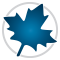Equation Solving - Maple Features - Maplesoft# Maple

Equation Solving

Maple can solve a wide range of equations and systems of equations. Maple employs different solving techniques:

• Symbolic methods for closed-form solutions.
• Numeric methods for approximate solutions.
• Hybrid symbolic and numeric algorithms for finding solutions to problems that are not solvable with purely symbolic or numeric methods.

Symbolic Methods for Exact, Closed-Form Solutions
Maple's symbolic solvers use state-of-the art algorithms for solving algebraic equations, including the F4 algorithm for computing Gröbner bases and a triangular set decomposition algorithm.

Maple allows you to:

• Solve equations and systems of equations.
• Solve inequalities and systems of inequalities.
• Find conditional solutions for many types of parametric equations and inequalities.
• Use ordinary variables or functions as unknowns. When the unknown is a function, Maple returns a function that solves the equation.
• Solve identities, parametric equations, non-linear systems, and series.
• Control the form of solutions.

Numeric Methods for Approximate Solutions
Maple's numeric solvers use industry-standard techniques for finding approximate solutions to equations, and include integrated solvers from the Numerical Algorithms Group (NAG).

Maple allows you to:

• Solve equations and systems of equations.
• Set the number of digits to be used in the computation.
• Specify a starting value.
• Specify an interval in which to search for solutions.
• Specify if you are looking for real or complex roots.
• Limit the number of solutions returned for a polynomial equation of one variable.
• Provide values to avoid during the search for solution, so those solutions will be ignored.

Hybrid Methods
Beyond simply applying standard numeric techniques, Maple extends the abilities and speed of its numeric solvers by applying a hybrid symbolic-numeric approach.

If a problem is in a form that cannot be solved by standard numerical or symbolic approaches, Maple attempts to transform the problem symbolically into an equivalent form, which is amenable to numerical methods.

Hybrid techniques are also employed to select appropriate starting values for numerical solvers, allowing them to arrive at an answer more quickly.

These hybrid approaches are fully integrated into the numeric solver algorithms and are applied automatically as needed.

Other Solvers
In addition to routines for algebraic equation solving, Maple has numerous specialized solvers including routines for differential equations, differential-algebraic equations, equations over the integers, equations over the integers mod m, recurrence equations, series solutions, and q-difference equations.

Prêt(e) pour l'étape suivante ?

*Ce programme d'évaluation n'est proposé actuellement ni aux étudiants ni aux particuliers.

Achetez: